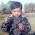Skip to main content

### How to renumber Columns in STAAD.Pro?

When we model the structure, the nodes, and elements gets a numerical identity. If these numbers are in sequence, it can be lot more easier to find the members faster result interpretation can be done. Speed of work can be increased if we know that the elements are numbered in sequential order. Here is a way to do so :

### For numbering columns as shown below.1. Select all columns of structure.
2. Go to geometry → renumber → members.
3. Press yes to the window that asks for confirmation.
4. Give first number from where you want to start your numbering of Columns.
5. Select available sort criteria in following sequence
• z coordinate
• x coordinate
• y coordinate
1. Click Accept.
2. You have done it.
3. If you want numbers in vertical sequence interchange x and z coordinate.

Read: How to renumber nodes in STAAD.Pro?

### Comments